pagerank python库_python pagerank - CSDN

• 原理参考链接：http://www.cnblogs.com/rubinorth/p/5799848.html 用到的程序： # -*- coding: utf-8 -*- """ Created on Wed May 03 10:53:32 2017 @author: ...from pygraph.classes.digraph import dig
原理参考链接：http://www.cnblogs.com/rubinorth/p/5799848.html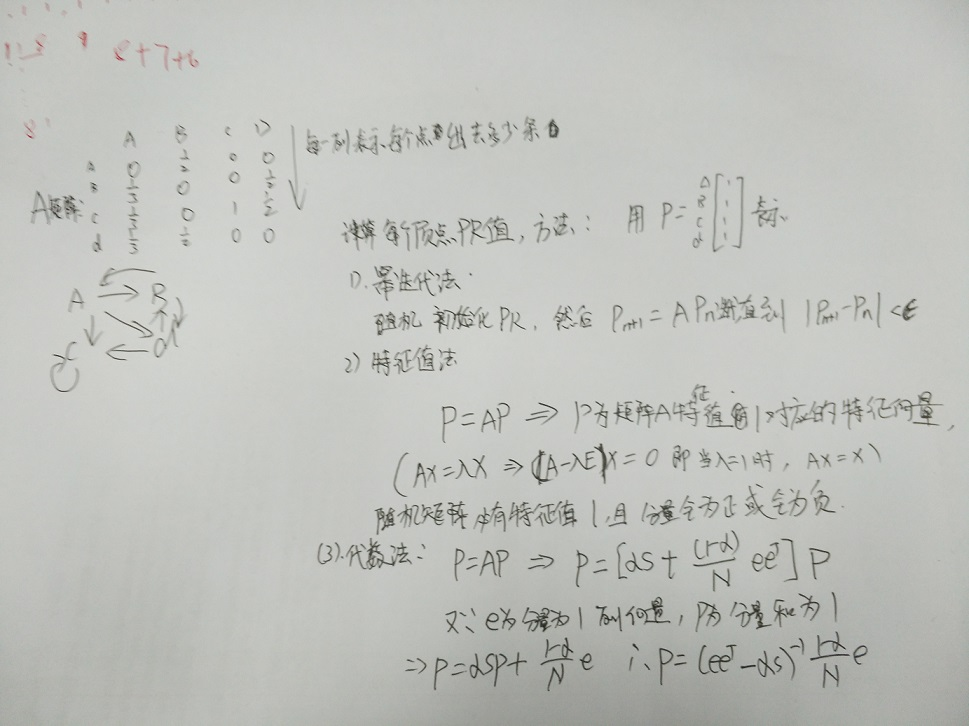用到的程序：

# -*- coding: utf-8 -*-
"""
Created on Wed May 03 10:53:32 2017

@author:
"""

from pygraph.classes.digraph import digraph

class PRIterator:
__doc__ = '''计算一张图中的PR值'''

def __init__(self, dg):
self.damping_factor = 0.85  # 阻尼系数,即α
self.max_iterations = 100  # 最大迭代次数
self.min_delta = 0.00001  # 确定迭代是否结束的参数,即ϵ
self.graph = dg

def page_rank(self):
#  先将图中没有出链的节点改为对所有节点都有出链
for node in self.graph.nodes():
if len(self.graph.neighbors(node)) == 0:
for node2 in self.graph.nodes():

nodes = self.graph.nodes()
graph_size = len(nodes)

if graph_size == 0:
return {}
page_rank = dict.fromkeys(nodes, 1.0 / graph_size)  # 给每个节点赋予初始的PR值
damping_value = (1.0 - self.damping_factor) / graph_size  # 公式中的(1−α)/N部分

flag = False
for i in range(self.max_iterations):
change = 0
for node in nodes:
rank = 0
for incident_page in self.graph.incidents(node):  # 遍历所有“入射”的页面
rank += self.damping_factor * (page_rank[incident_page] / len(self.graph.neighbors(incident_page)))
rank += damping_value
change += abs(page_rank[node] - rank)  # 绝对值
page_rank[node] = rank

print("This is NO.%s iteration" % (i + 1))
print(page_rank)

if change < self.min_delta:
flag = True
break
if flag:
print("finished in %s iterations!" % node)
else:
print("finished out of 100 iterations!")
return page_rank

if __name__ == '__main__':
dg = digraph()

pr = PRIterator(dg)
page_ranks = pr.page_rank()

print("The final page rank is\n", page_ranks)

需要安装工具包python-graph-core：https://github.com/pmatiello/python-graph

教训：一开始直接pip安装了pygraph,发现不行《然后在github上下载下来，用python setup.py install 安装，将程序拷贝到该安装目录下，发现程序还是运行不了，然后讲pygraph卸载后就可以了，但是程序放在其他地方运行不了，然后用spyder运行也是无法运行,重启下解决


展开全文python pagerank
• PageRank有点被神化了，其实公式很简单。 文章目录简述算法模型定义Flow版本Google Formula实现 算法 主要是分为两种： The ‘Flow’ formula The Google formula 模型定义 很多个网页，直接存在链路关系，设为G，...
简述
PageRank有点被神化了，其实公式很简单。
算法
主要是分为两种：

The ‘Flow’ formula

模型定义
很多个网页，直接存在链路关系，设为G，N*N的矩阵
这里先只考虑有向无权无环图，即边有方向，且权重都一样，且没有自己到自己的边（环）。

N为节点数或者是网页数
G[i][j] = 1表示，i->j有条边

Flow版本
$r_i = \sum_{(j=1) and (j -> i)}^N{\frac{r_j}{d_j}}$
一个很好是数学写法就是：
$r = M * r$

如果有i->j, M[j][i] = 1/dj，否者为0

算法流程：

也就是，随机初始化每个点的分数

然后，迭代：

每个点的分数，由所有 指向他的节点的分数 除以 这个节点的出度数 求和所替代

Flow版本会出现两个重要的问题;

Dead End：例如只有两个点，然后边为A->B，B就是一个Dead END。即到这时候的分数就出不去了。
Spider Traps：循环指向，例如A->B->A。那么这个分数就会这之间打转

提出了一个叫做Teleport的概念，也称之为意念转移。

$r_i = \beta * \sum_{(j=1) and (j -> i)}^N{\frac{r_j}{d_j}} + (1 - \beta) * \frac{1}{N}$
直接的数学表达：
$r = A * r \\ A = \beta * M + (1-\beta) * [\frac{1}{N}]_{N*N}$
$r = \beta * M * r + [\frac{1}{N}]_N$

如果有i->j, M[j][i] = 1/dj，否者为0

存在的问题：

虽然直接的数学表达会更简洁，但是多出来的A一定会是稠密矩阵使得空间消耗为O(N^2)了，这样的在网站达到数亿的情况下，这个东西就不太现实了。

实现
实现同样做两个不同的版本的：
但有些共同的模型：
导入包：
import numpy as np
import random

创造数据：
def create_data(N, alpha=0.5): # random > alpha, then here is a edge.
G = np.zeros((N, N))
for i in range(N):
for j in range(N):
if i == j:
continue
if random.random() < alpha:
G[i][j] = 1
return G
G = create_data(10)

GtoM：
def GtoM(G, N):
M = np.zeros((N, N))
for i in range(N):
D_i = sum(G[i])
if D_i == 0:
continue
for j in range(N):
M[j][i] = G[i][j] / D_i # watch out! M_j_i instead of M_i_j
return M
M = GtoM(G, 10)


Flow版本的PageRank

def PageRank(M, N, T=300, eps=1e-6):
R = np.ones(N) / N
for time in range(T):
R_new = np.dot(M, R)
if np.linalg.norm(R_new - R) < eps:
break
R = R_new.copy()
return R_new

测试下：
values = PageRank(M, 10, T=2000)
values

输出：
array([0.09972576, 0.09193927, 0.07843151, 0.09125886, 0.08925602,
0.10407245, 0.09623654, 0.13851257, 0.13086464, 0.07970237])


def PageRank(M, N, T=300, eps=1e-6, beta=0.8):
R = np.ones(N) / N
teleport = np.ones(N) / N
for time in range(T):
R_new = beta * np.dot(M, R) + (1-beta)*teleport
if np.linalg.norm(R_new - R) < eps:
break
R = R_new.copy()
return R_new

同样的数据测试下：
测试下：
values = PageRank(M, 10, T=2000)
values

输出：
array([0.09815807, 0.09250429, 0.08376235, 0.09300133, 0.09324628,
0.10108776, 0.09855127, 0.13019363, 0.12458992, 0.0849051 ])



展开全文PageRank Python 数据挖掘
• .PageRank算法--从原理到实现   零. PageRank算法简介 PageRank算法，即网页排名算法，由Google创始人Larry Page在斯坦福上学的时候提出来的。该算法用于对网页进行排名，排名高的网页表示该网页被访问的概率高...
参考

.PageRank算法--从原理到实现

零. PageRank算法简介

该算法的主要思想有两点：

a. 如果多个网页指向某个网页A，则网页A的排名较高。

b. 如果排名高A的网页指向某个网页B，则网页B的排名也较高，即网页B的排名受指向其的网页的排名的影响。

一、PageRank算法原理

1. 简单的PageRank算法

如图是一个4个网页之间的链接情况：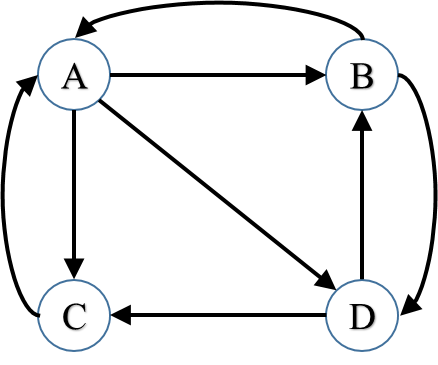假设网页X的排名用PR(X)表示，则A的排名为PR(A)，由图可知，网页B和C指向了网页A，那么网页A的排名可以表示为：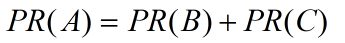网页C只指向了A，不指向其他网页，然而网页B不仅指向了A，还指向了D，因此上面的公式更合理地修改为：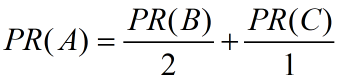意思是，B的PageRank值被分给了A和D，而C的PageRank值全都给了A。

有的网页，没有指向其他网页，如下图中的C网页。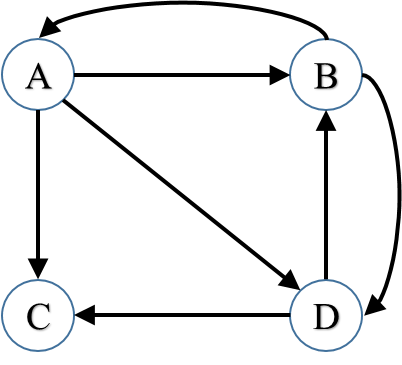那么，假设网页C的PageRank值被均分为到图中的所有网页（4个网页），那么A网页的PageRank值可以表示为：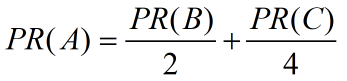3. 网页链接中存在环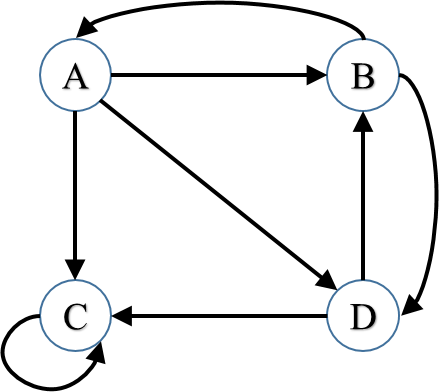图中网页C指向网页C，不指向其他网页。现实中，网页自己指向自己的情况可能不太常见，但是有可能的情况是：若干个页面形成一个环，那么用户在进入其中某个网页的时候，就陷入这个循环中。

假设当一个用户，遇上这种情况时，以某个概率α随机指向其他任意一个网页，每个网页的概率相等。因此上图中的网页A的PageRank值可以表示为：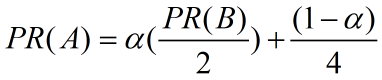上面这个公式可以解释为：α表示用户从网页B以概率α链接到网页A，后面的（1-α）表示用户从网页C以概率（1-α）链接到网页A。

即：

网页B的PageRank值分配情况为：α*1/2给A, α*1/2给D,(1-α)/4分别给4个网页。

网页C的PageRank值分配情况为：α*1给自己C（1-α）*1/4分别给其他网页。

4. 更一般的PageRank公式

综合上面的论述，一般的PageRank计算公式为：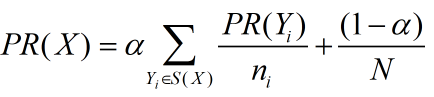其中S(X)表示，指向网页X的所有网页的集合，n_i表示网页Y_i的出边数量，N表示所有网页总数，α一般取0.85。

二、PageRank值的计算方法

1. 迭代法

利用前面得到的公式，进行迭代，直到迭代前后两次的差值在允许的阈值范围内，迭代结束。

当然，可以将迭代过程写成矩阵形式。推导过程如下：

针对前面的最后一个网络图，可以分别得到各个网页的PageRank值得计算公式，如下：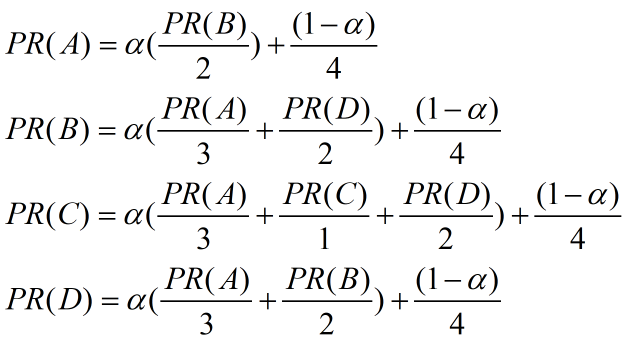写成矩阵的形式为：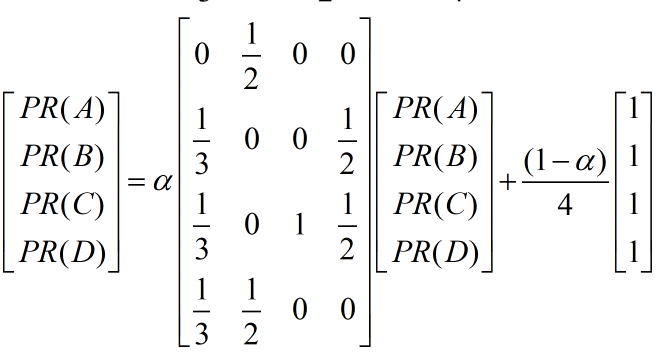可以将上面的列向量和矩阵分别记为一些符号，上式表示为：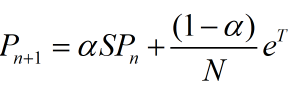还有更简洁的记法，记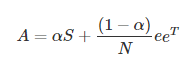A是一个常数矩阵，那么，就有迭代公式：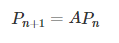也可以根据这个公式，进行迭代。

2. 代数法

因为，PageRank算法最终收敛（这个结论可以证明，此文不证明），因此，收敛时刻的PageRank值组成的列向量P应当满足：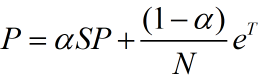因此有：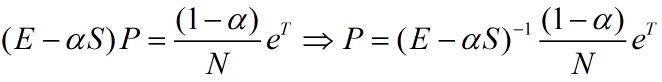这个方法不用迭代，求出矩阵的逆，就可以求出PageRank值组成的列向量P（然而，计算大规模的矩阵的逆，也是个难题。因此，这个方法代码简单，但效率可能不如迭代方法高）

三、Python实现

下面仅仅实现迭代法，代码如下，需要用到Python的numpy库用于矩阵乘法：

# 输入为一个*.txt文件，例如
# A B
# B C
# B A
# ...表示前者指向后者

import numpy as np

if __name__ == '__main__':

# 读入有向图，存储边
f = open('input_1.txt', 'r')
edges = [line.strip('\n').split(' ') for line in f]
print(edges)

# 根据边获取节点的集合
nodes = []
for edge in edges:
if edge not in nodes:
nodes.append(edge)
if edge not in nodes:
nodes.append(edge)
print(nodes)

N = len(nodes)

# 将节点符号（字母），映射成阿拉伯数字，便于后面生成A矩阵/S矩阵
i = 0
node_to_num = {}
for node in nodes:
node_to_num[node] = i
i += 1
for edge in edges:
edge = node_to_num[edge]
edge = node_to_num[edge]
print(edges)

# 生成初步的S矩阵
S = np.zeros([N, N])
for edge in edges:
S[edge, edge] = 1
print(S)

# 计算比例：即一个网页对其他网页的PageRank值的贡献，即进行列的归一化处理
for j in range(N):
sum_of_col = sum(S[:,j])
for i in range(N):
S[i, j] /= sum_of_col
print(S)

# 计算矩阵A
alpha = 0.85
A = alpha*S + (1-alpha) / N * np.ones([N, N])
print(A)

# 生成初始的PageRank值，记录在P_n中，P_n和P_n1均用于迭代
P_n = np.ones(N) / N
P_n1 = np.zeros(N)

e = 100000  # 误差初始化
k = 0   # 记录迭代次数
print('loop...')

while e > 0.00000001:   # 开始迭代
P_n1 = np.dot(A, P_n)   # 迭代公式
e = P_n1-P_n
e = max(map(abs, e))    # 计算误差
P_n = P_n1
k += 1
print('iteration %s:'%str(k), P_n1)

print('final result:', P_n)

输入的input_1.txt文本内容为：

A B
A C
A D
B D
C E
D E
B E
E A

结果为：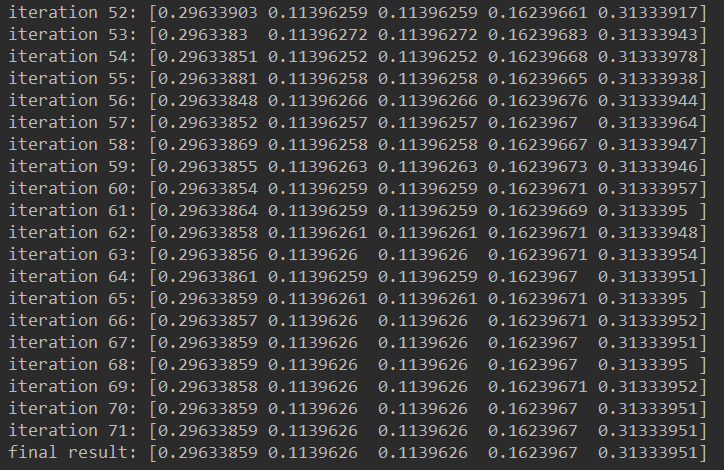最后的一个数组，分别为A, B, C, D, E的PageRank值，其中E最高， A第二高， B和C相同均最低。

我们再来看一下这个可视化的有向图：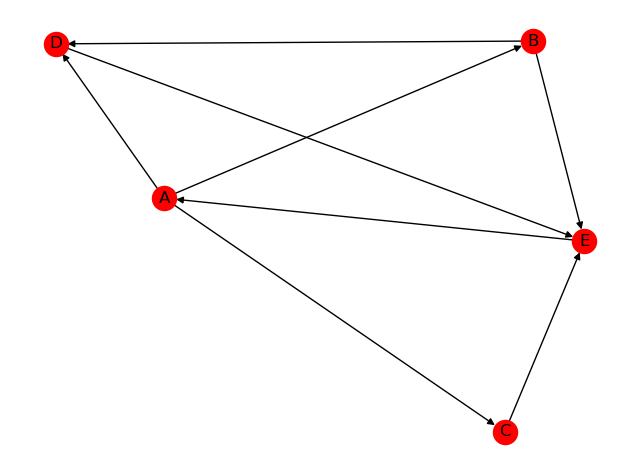可以看出，有3条边指向E。再看，指向A的这个点是E点，因此A的PageRank值也很高，可以说“A沾了E的光”。

上面的可视化代码如下：

import networkx as nx
import matplotlib.pyplot as plt

if __name__ == '__main__':

# 读入有向图，存储边
f = open('input_1.txt', 'r')
edges = [line.strip('\n').split(' ') for line in f]

G = nx.DiGraph()
for edge in edges:
nx.draw(G, with_labels=True)
plt.show()



展开全文PageRank 算法 经典算法
• Python NetworkxPageRank算法实现源码分析 网上对Page算法讲解的很多，实现代码也很多很杂， 所以为了找到一个更高质量的PageRank算法的实现， 我阅读了Python Networkx上自带的pagerank方法的源码。...

网上对Page算法讲解的很多，实现代码也很多很杂， 所以为了找到一个更高质量的PageRank算法的实现，
我阅读了Python Networkx库上自带的pagerank方法的源码。部分多余内容我删除了，有兴趣可以直接下这个库查看源码
具体的pagerank代码我已经上传到网盘在http://pan.baidu.com/s/1ntOafH3

PageRank算法最主要的地方在于对两个问题的解决，一个是dangling nodes，一个是spider trap
前者是说，没有引用其他网页的链接，用图角度的理解就是出度为0。
后者是说，进入到一个网页或者几个网页，这个单个网页，或者几个网页之间相互引用，这样资源进去后就一直在里面转，出不来了，造成rank sink问题。
对于dangling nodes，我们可以计算他们的PR贡献值,然后均分给所有节点
对于spider trap，需要用心灵漂移的方式去解决。
Networkx库里面，主要有三种计算PageRank的方法（PS为什么是三种，我直观觉得是因为这是三个人写的，因为连某些效果完全相同的初始化语句，三个写的都不一样）
1 pagerank函数
以图结构为基础
通过迭代收敛方法计算PR值(PageRank值)
2 pagerank_numpy函数
将图转换为numpy邻接矩阵，
通过计算最大特征值对应的主特征向量，即为所求PR值
3 pagerank_scipy函数
将图转换为sparse稀疏矩阵
通过迭代收敛方法计算PR值

"""PageRank analysis of graph structure. """
#    NetworkX:http://networkx.lanl.gov/
import networkx as nx

@not_implemented_for('multigraph')
def pagerank(G, alpha=0.85, personalization=None,
max_iter=100, tol=1.0e-6, nstart=None, weight='weight',
dangling=None):
"""Return the PageRank of the nodes in the graph.
Parameters
-----------
G : graph
A NetworkX graph. 在PageRank算法里面是有向图
alpha : float, optional
稳定系数, 默认0.85, 心灵漂移teleporting系数，用于解决spider trap问题
personalization: dict, optional
个性化向量，确定在分配中各个节点的权重
格式举例，比如四个点的情况: {1:0.25,2:0.25,3:0.25,4:0.25}
默认个点权重相等，也可以给某个节点多分配些权重，需保证权重和为1.
max_iter : integer, optional
最大迭代次数
tol : float, optional
迭代阈值
nstart : dictionary, optional
整个网络各节点PageRank初始值
weight : key, optional
各边权重

dangling: dict, optional
字典存储的是dangling边的信息
key   --dangling边的尾节点，也就是dangling node节点
value --dangling边的权重
PR值按多大程度将资源分配给dangling node是根据personalization向量分配的
This must be selected to result in an irreducible transition
matrix (see notes under google_matrix). It may be common to have the
dangling dict to be the same as the personalization dict.

Notes
-----
特征值计算是通过迭代方法进行的，不能保证收敛，当超过最大迭代次数时，还不能减小到阈值内，就会报错

"""

#步骤一：图结构的准备--------------------------------------------------------------------------------
if len(G) == 0:
return {}

if not G.is_directed():
D = G.to_directed()
else:
D = G

# Create a copy in (right) stochastic form
W = nx.stochastic_graph(D, weight=weight)
N = W.number_of_nodes()

# 确定PR向量的初值
if nstart is None:
x = dict.fromkeys(W, 1.0 / N)  #和为1
else:
# Normalized nstart vector
s = float(sum(nstart.values()))
x = dict((k, v / s) for k, v in nstart.items())

if personalization is None:
# Assign uniform personalization vector if not given
p = dict.fromkeys(W, 1.0 / N)
else:
missing = set(G) - set(personalization)
if missing:
raise NetworkXError('Personalization dictionary '
'must have a value for every node. '
'Missing nodes %s' % missing)
s = float(sum(personalization.values()))
p = dict((k, v / s) for k, v in personalization.items()) #归一化处理

if dangling is None:
# Use personalization vector if dangling vector not specified
dangling_weights = p
else:
missing = set(G) - set(dangling)
if missing:
raise NetworkXError('Dangling node dictionary '
'must have a value for every node. '
'Missing nodes %s' % missing)
s = float(sum(dangling.values()))
dangling_weights = dict((k, v/s) for k, v in dangling.items())

dangling_nodes = [n for n in W if W.out_degree(n, weight=weight) == 0.0]

#dangling_nodes  dangling节点
#danglesum       dangling节点PR总值

#dangling初始化  默认为personalization
#dangling_weights  根据dangling而生成，决定dangling node资源如何分配给全局的矩阵

# 迭代计算--------------------------------------------------------------------

#PR=alpha*(A*PR+dangling分配)+(1-alpha)*平均分配
#也就是三部分，A*PR其实是我们用图矩阵分配的，dangling分配则是对dangling node的PR值进行分配，(1-alpha)分配则是天下为公大家一人一份分配的

#其实通俗的来说，我们可以将PageRank看成抢夺大赛，有三种抢夺机制。
#1，A*PR这种是自由分配，大家都愿意参与竞争交流的分配
#2，dangling是强制分配，有点类似打倒土豪分田地的感觉，你不参与自由市场，那好，我们就特地帮你强制分。
#3，平均分配，其实就是有个机会大家实现共产主义了，不让spider trap这种产生rank sink的节点捞太多油水，其实客观上也是在帮dangling分配。

#从图和矩阵的角度来说，可以这样理解，我们这个矩阵可以看出是个有向图
#矩阵要收敛-->矩阵有唯一解-->n阶方阵对应有向图是强连通的-->两个节点相互可达，1能到2,2能到1
#如果是个强连通图，就是我们上面说的第1种情况，自由竞争，那么我们可以确定是收敛的
#不然就会有spider trap造成rank sink问题

for _ in range(max_iter):
xlast = x
x = dict.fromkeys(xlast.keys(), 0)  #x初值
danglesum = alpha * sum(xlast[n] for n in dangling_nodes) #第2部分：计算dangling_nodes的PR总值
for n in x:
for nbr in W[n]:
x[nbr] += alpha * xlast[n] * W[n][nbr][weight]    #第1部分:将节点n的PR资源分配给各个节点，循环之
for n in x:
x[n] += danglesum * dangling_weights[n] + (1.0 - alpha) * p[n]   #第3部分：节点n加上dangling nodes和均分的值

# 迭代检查
err = sum([abs(x[n] - xlast[n]) for n in x])
if err < N*tol:
return x
raise NetworkXError('pagerank: power iteration failed to converge '
'in %d iterations.' % max_iter)

nodelist=None, weight='weight', dangling=None):
"""Return the Google matrix of the graph.

Parameters
-----------
和PageRank参数表相似

Returns
-------
A : NumPy matrix

Notes
-----

这个方法返回的“谷歌矩阵” 描述了PageRank用到的马尔科夫链。
PageRank需要收敛的话，就需要方程有唯一解，这样过度矩阵必须是不可約的。
用图的角度讲，就是说两个点之间必须相互可达，节点1能到节点2,节点2也有路径到1。
不然的话，会出现spider trap，导致rank sink问题出现，造成矩阵无法收敛，集中到sink节点了。

The matrix returned represents the transition matrix that describes the
Markov chain used in PageRank. For PageRank to converge to a unique
solution (i.e., a unique stationary distribution in a Markov chain), the
transition matrix must be irreducible. In other words, it must be that
there exists a path between every pair of nodes in the graph, or else there
is the potential of "rank sinks."

"""
import numpy as np

if nodelist is None:
nodelist = G.nodes()

M = nx.to_numpy_matrix(G, nodelist=nodelist, weight=weight)
N = len(G)
if N == 0:
return M

# Personalization vector
if personalization is None:
p = np.repeat(1.0 / N, N)
else:
missing = set(nodelist) - set(personalization)
if missing:
raise NetworkXError('Personalization vector dictionary '
'must have a value for every node. '
'Missing nodes %s' % missing)
p = np.array([personalization[n] for n in nodelist], dtype=float)
p /= p.sum()

# Dangling nodes
if dangling is None:
dangling_weights = p
else:
missing = set(nodelist) - set(dangling)
if missing:
raise NetworkXError('Dangling node dictionary '
'must have a value for every node. '
'Missing nodes %s' % missing)
# 确定dangling node的PR资源的分配矩阵
dangling_weights = np.array([dangling[n] for n in nodelist],dtype=float)
dangling_weights /= dangling_weights.sum()

dangling_nodes = np.where(M.sum(axis=1) == 0)  #计算行和为0，也就是计算出度为0的节点，就是dangling nodes

# Assign dangling_weights to any dangling nodes (nodes with no out links)
for node in dangling_nodes:
M[node] = dangling_weights    #将这个的出度直接设成平均分配

M /= M.sum(axis=1)  # 归一化

#这个时候M矩阵已经解决了dangling node的问题，再通过1-alpha解决
return alpha * M + (1 - alpha) * np.outer(np.ones(N), p)

def pagerank_numpy(G, alpha=0.85, personalization=None, weight='weight',
dangling=None):
"""
备注
特征向量计算，是利用了Numpy在LAPACK库的方法进行的，对小型图的计算非常快速精确
"""
import numpy as np
if len(G) == 0:
return {}
weight=weight, dangling=dangling)
# use numpy LAPACK solver
eigenvalues, eigenvectors = np.linalg.eig(M.T)
ind = eigenvalues.argsort()
# eigenvector of largest eigenvalue at ind[-1], normalized
largest = np.array(eigenvectors[:, ind[-1]]).flatten().real
norm = float(largest.sum())
return dict(zip(G, map(float, largest / norm)))

def pagerank_scipy(G, alpha=0.85, personalization=None,
max_iter=100, tol=1.0e-6, weight='weight',
dangling=None):
"""
-----
该方法应用的是SciPy库，实现方法和第一个：pagerank函数是基本一致的

-----
备注
特征向量计算，是利用了SciPy库稀疏矩阵计算
"""
import scipy.sparse

N = len(G)
if N == 0:
return {}

nodelist = G.nodes()
M = nx.to_scipy_sparse_matrix(G, nodelist=nodelist, weight=weight,
dtype=float)
S = scipy.array(M.sum(axis=1)).flatten()
S[S != 0] = 1.0 / S[S != 0]
Q = scipy.sparse.spdiags(S.T, 0, *M.shape, format='csr')
M = Q * M

# 初始化PageRank值
x = scipy.repeat(1.0 / N, N)

# 确定personalization字典，各节点分配权重，默认权重相同
if personalization is None:
p = scipy.repeat(1.0 / N, N)
else:
missing = set(nodelist) - set(personalization)
if missing:
raise NetworkXError('Personalization vector dictionary '
'must have a value for every node. '
'Missing nodes %s' % missing)
p = scipy.array([personalization[n] for n in nodelist],
dtype=float)
p = p / p.sum()

# Dangling nodes的确定和资源配置
if dangling is None:
dangling_weights = p
else:
missing = set(nodelist) - set(dangling)
if missing:
raise NetworkXError('Dangling node dictionary '
'must have a value for every node. '
'Missing nodes %s' % missing)
# Convert the dangling dictionary into an array in nodelist order
dangling_weights = scipy.array([dangling[n] for n in nodelist],
dtype=float)
dangling_weights /= dangling_weights.sum()
is_dangling = scipy.where(S == 0)

# 迭代
#第1部分:x*M, 第2部分dangling node分配, 第3部分rank sink解决
for _ in range(max_iter):
xlast = x
x = alpha * (x * M + sum(x[is_dangling]) * dangling_weights) + (1 - alpha) * p
# check convergence, l1 norm
err = scipy.absolute(x - xlast).sum()
if err < N * tol:
return dict(zip(nodelist, map(float, x)))
raise NetworkXError('pagerank_scipy: power iteration failed to converge '
'in %d iterations.' % max_iter)

展开全文• ## 初学python书籍推荐

万次阅读 多人点赞 2018-05-04 13:50:42
• 机器学习实战 ... 本系列博客源自于读《机器学习实战—中文版》这本书的学习笔记，用于日后翻阅、查看资料用。 机器学习算法越来越受到人们的青睐，是由于这些算法在一定程度上可以达到智能的目的，比如人脸识别、...机器学习 机器学习实战 python
• ## 初学python，看这些书就够了！

万次阅读 多人点赞 2016-12-15 11:22:52
迎接大数据浪潮，大家可以从大数据技术的教学书籍上下手，早日脱离苦海，今天我们大圣众包小编继续为大家精选几本python的书籍！ 《Python学习手册（第4版）》 　【内容介绍】通过《Python学习...大数据 python 机器学习 开发人员 网络编程
• 2010 年 5 月 5 日更新：我翻译了一篇《非死不可》作为对本文观点的进一步支持和对评论的回复，请见：http://blog.csdn.net/lanphaday/archive/2010/05/05/5558617.aspx为什么《Dive into Python》不值得推荐作者：...python decorator 正则表达式 性能优化 单元测试
• 使用TextRank提取关键字 ...每个单词作为pagerank中的一个节点。设定窗口大小为k，假设一个句子依次由下面的单词组成： w1, w2, w3, w4, w5, ..., wn w1,w2, ..., wk、w2, w3, ...,wk+1、w3,文本聚类
• PageRank算法原理与Python实现   参考 .PageRank算法--从原理到实现   零. PageRank算法简介 PageRank算法，即网页排名算法，由Google创始人Larry Page在斯坦福上学的时候提出来的。该算法用于对网页进行...
• 本文源代码转自搜索引擎原理，博主进行整理调BUG并进行注释，对于Python初学者来说是了解爬虫、网页排序算法非常好的素材。 首先来介绍一下PageRank网页排序算法（注：转自PageRank算法简介及Map-Reduce实现，详情...python 搜索引擎 排序算法 爬虫
• Pyhusky Operators PyHusky支持三种运算符： Load, Transformation, 和 Action. 通畅情况下，一个PyHusky程序首先使用Load运算符. PyHusky 采用lazy evaluation技术, 所有 Loads 和 Transformations 的...大数据
• ## python爬虫的原理介绍

万次阅读 多人点赞 2019-06-24 21:04:57
一、爬虫与数据 （一）为什么要做爬虫 都说现在是大数据时代，但是与之相对应的问题是，大数据中的数据从何而来。可以人工收集数据，但是人工收集数据的效率却免不了太过低下。也可以找一些专门从事数据服务的公司...TextRank PageRank 关键词提取
• ## Django 网页框架

千次阅读 2013-02-20 22:46:47
小白在经历过写通讯录软件、网页服务和手机APP后，对Python的应用已经非常熟悉了。 小白琢磨着，自己已经快毕业了，何不用Python来帮助自己创业呢？用Python做些什么好呢？小白曾经给EPTCO Inc. 做过搜索引擎优化和...python Python
• 大数据与人工智能时代，掌握Python基础后，我们可以选择数据分析方向、人工智能方向、全栈开发方向...如果想要追赶 Python 的热潮，应该如何学习呢？除了自学之外，多数人都会选择在线课程作为辅助。选择课程的衡量...networkx
• ## python 中文分词工具

千次阅读 2018-06-24 16:30:03
python 中文分词工具 jieba，https://github.com/fxsjy/jieba jieba_fast，https://github.com/deepcs233/jieba_fast nltk，https://github.com/nltk/nltk FoolNLTK，...python 中文分词 工具
• 1.数据分类 按数据形态，我们把数据分为结构化数据和非结构化数据两种。 结构化数据如传统的Data Warehouse数据，字段有固定的长度和语义，计算机程序可以直接处理 非结构化数据有文本数据、图像数据、自然语言数据...
...